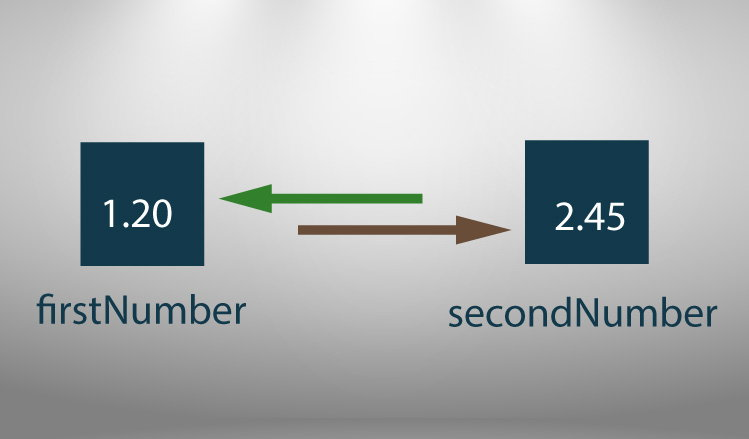# C Program to Swap Two Numbers

This example contains two different techniques to swap numbers in C programming. The first program uses temporary variable to swap numbers, whereas the second program doesn't use temporary variables.To understand this example, you should have the knowledge of following C programming topics:

## Example 1: Program to Swap Numbers Using Temporary Variable

``````#include <stdio.h>
int main()
{
double firstNumber, secondNumber, temporaryVariable;

printf("Enter first number: ");
scanf("%lf", &firstNumber);

printf("Enter second number: ");
scanf("%lf",&secondNumber);

// Value of firstNumber is assigned to temporaryVariable
temporaryVariable = firstNumber;

// Value of secondNumber is assigned to firstNumber
firstNumber = secondNumber;

// Value of temporaryVariable (which contains the initial value of firstNumber) is assigned to secondNumber
secondNumber = temporaryVariable;

printf("\nAfter swapping, firstNumber = %.2lf\n", firstNumber);
printf("After swapping, secondNumber = %.2lf", secondNumber);

return 0;
}
```
```

Output

```Enter first number: 1.20
Enter second number: 2.45

After swapping, firstNumber = 2.45
After swapping, secondNumber = 1.20
```

In the above program, the temporaryVariable is assigned the value of firstNumber.
Then, the value of firstNumber is assigned to secondNumber.
Finally, the temporaryVariable (which holds the initial value of firstNumber) is assigned to secondNumber which completes the swapping process.

Here, temporaryVariable is used to hold the value of firstNumber and doesn't have any other use except that. You can also write the swapping program without using temporaryVariable.

## Example 2: Program to Swap Number Without Using Temporary Variables

``````#include <stdio.h>
int main()
{
double firstNumber, secondNumber;

printf("Enter first number: ");
scanf("%lf", &firstNumber);

printf("Enter second number: ");
scanf("%lf",&secondNumber);

// Swapping process

firstNumber = firstNumber - secondNumber;
secondNumber = firstNumber + secondNumber;
firstNumber = secondNumber - firstNumber;

printf("\nAfter swapping, firstNumber = %.2lf\n", firstNumber);
printf("After swapping, secondNumber = %.2lf", secondNumber);

return 0;
}``````

Output

```Enter first number: 10.25
Enter second number: -12.5

After swapping, firstNumber = -12.50
After swapping, secondNumber = 10.25```